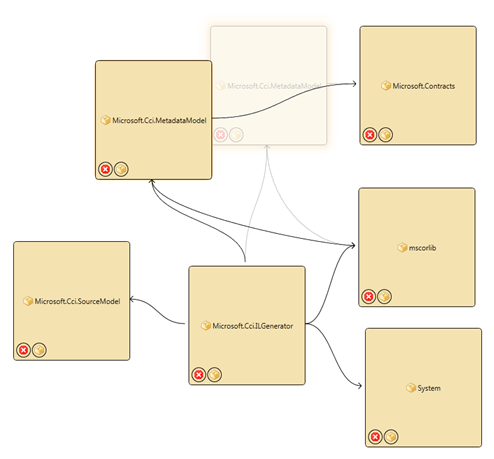WPF or Silverlight PathBuilder

Every once in a while you find yourself needing to draw some type of a shape dynamically. One easy example is arrows connecting visuals that are movable. In some cases you can simply place arrows where you expect the visuals to be but in others the visuals can move around in non-fixed ways. For those scenarios you need to create lines dynamically.In the composite screenshots above I am showing a scenario where I am able to drag the nodes around free form and I need the arrows to continuously update as the visual is moving to show relationships. You can imagine various other scenarios where you would need data driven shapes.

I have created a simple API for creating Path data in WPF or Silverlight. It is based on the idea that you can bind a string to the Data property of a Path object and all you need to do is to produce valid Path Data syntax in a string and you get a dynamic shape.

public enum SweepDirection
{
Clockwise,
CounterClockwise
}

public interface IPath
{
string Data { get;}
}

public static class PathBuilder
{
private enum DrawCommand
{
Start,
Move,
Line,
HorizontalLine,
VerticalLine,
CubicBezierCurve,
SmoothCubicBezierCurve,
EllipticalArc,
Close
}

public static IPath Start()
{
return new Path(DrawCommand.Start, "");
}

private class Path : IPath
{
private DrawCommand lastCommand = DrawCommand.Start;
private string data;
public Path(DrawCommand command, string data)
{
this.lastCommand = command;
this.data = data;
}

public string Data
{
get { return this.data; }
}

public DrawCommand Command
{
get { return this.lastCommand; }
}
}

public static IPath Move(this IPath path, Point p)
{
var prefix = PathBuilder.AppendCommandPrefix(((Path)path).Command, DrawCommand.Move);
return new Path(
DrawCommand.Move,
path.Data + prefix + string.Format(" {0} {1}", p.X, p.Y));
}

public static IPath DrawLine(this IPath path, Point end)
{
var prefix = AppendCommandPrefix(((Path)path).Command, DrawCommand.Line);
return new Path(
DrawCommand.Line,
path.Data + prefix + string.Format(" {0} {1}", end.X, end.Y));
}

public static IPath DrawHorizontalLine(this IPath path, double x)
{
var prefix = AppendCommandPrefix(((Path)path).Command, DrawCommand.HorizontalLine);
return new Path(
DrawCommand.HorizontalLine,
path.Data + prefix + string.Format(" {0}", x));
}

public static IPath DrawVerticalLine(this IPath path, double y)
{
var prefix = AppendCommandPrefix(((Path)path).Command, DrawCommand.VerticalLine);
return new Path(
DrawCommand.VerticalLine,
path.Data + prefix + string.Format(" {0}", y));
}

public static IPath DrawCubicBezierCurve(this IPath path, Point controlPoint1, Point controlPoint2, Point end)
{
var prefix = AppendCommandPrefix(((Path)path).Command, DrawCommand.CubicBezierCurve);
return new Path(
DrawCommand.CubicBezierCurve,
path.Data + prefix + string.Format(" {0} {1} {2} {3} {4} {5}",
controlPoint1.X,
controlPoint1.Y,
controlPoint2.X,
controlPoint2.Y,
end.X,
end.Y));
}

public static IPath DrawQuadraticBezierCurve(this IPath path, Point controlPoint, Point end)
{
return new Path(
path.Data + prefix + string.Format(" {0} {1} {2} {3}",
controlPoint.X,
controlPoint.Y,
end.X,
end.Y));
}

public static IPath DrawSmoothCubicBezierCurve(this IPath path, Point controlPoint, Point end)
{
var prefix = AppendCommandPrefix(((Path)path).Command, DrawCommand.SmoothCubicBezierCurve);
return new Path(DrawCommand.SmoothCubicBezierCurve,
path.Data + prefix + string.Format(" {0} {1} {2} {3}",
controlPoint.X,
controlPoint.Y,
end.X,
end.Y));
}

public static IPath DrawSmoothQuadraticBezierCurve(this IPath path, Point controlPoint, Point end)
{
return new Path(
path.Data + prefix + string.Format(" {0} {1} {2} {3}",
controlPoint.X,
controlPoint.Y,
end.X,
end.Y));
}

public static IPath DrawEllipticalArc(this IPath path, Size size, double rotationAngle, bool isLargeArc, SweepDirection sweepDirection, Point end)
{
var prefix = AppendCommandPrefix(((Path)path).Command, DrawCommand.EllipticalArc);
return new Path(
DrawCommand.EllipticalArc,
path.Data + prefix + string.Format(" {0} {1} {2} {3} {4} {5} {6}",
size.Width,
size.Height,
rotationAngle,
isLargeArc ? 1 : 0,
sweepDirection == SweepDirection.Clockwise ? 0 : 1,
end.X,
end.Y));
}

public static IPath Close(this IPath path)
{
var prefix = AppendCommandPrefix(((Path)path).Command, DrawCommand.Close);
return new Path(DrawCommand.Close, path.Data + prefix);
}

private static string AppendCommandPrefix(DrawCommand last, DrawCommand command)
{
if (last != command)
{
char c;
switch (command)
{
case DrawCommand.Move:
if (last == DrawCommand.Move)
throw new InvalidOperationException("Cannot have two move commands in a row.");

c = 'M';
break;
case DrawCommand.Line:
c = 'L';
break;
case DrawCommand.HorizontalLine:
c = 'H';
break;
case DrawCommand.VerticalLine:
c = 'V';
break;
case DrawCommand.CubicBezierCurve:
c = 'C';
break;
c = 'Q';
break;
case DrawCommand.SmoothCubicBezierCurve:
c = 'S';
break;
c = 'T';
break;
case DrawCommand.EllipticalArc:
c = 'A';
break;
case DrawCommand.Close:
if (last == DrawCommand.Close)
throw new InvalidOperationException("Cannot have two Close commands in a row.");

c = 'Z';
break;
default:
throw new NotSupportedException();
}

return string.Format(CultureInfo.InvariantCulture, " {0}", c);
}

return string.Empty;
}
}

Here is a snippet for drawing the arrows like I am doing above:

var pathData = PathBuilder.Start()
.Move(start)
.DrawCubicBezierCurve(cp1, cp2, end)
.Move(end)
.DrawLine(ap1)
.Move(end)
.DrawLine(ap2)
.Data;

It’s a fluent interface that returns an immutable IPath for each draw call so you can reuse parts of paths and branch shapes without having to redraw the entire thing every time. I will leave it up to you to figure out where to put all of your points but the above snippet draws an arrow.

I am doing this code in my ViewModel in a string Property based on the state of my model and I am actually rendering it by Binding that to the Data property on a Path object. The related XAML snippet looks like this:

<Path
Data="{Binding PathData}"
Stroke="Black"
StrokeThickness="1" />

As strange as it seems the Data property can accept a string and when bound will redraw the path as the bound property changes.Author: justinmchase

I'm a Software Developer from Minnesota.

Posted on Categories ProgrammingTags , ,